# Question 16 (Mandatory) (4.256 points) Carbonyl bromide decomposes to carbon monoxide and bromine. COBr2(g) = CO(g)...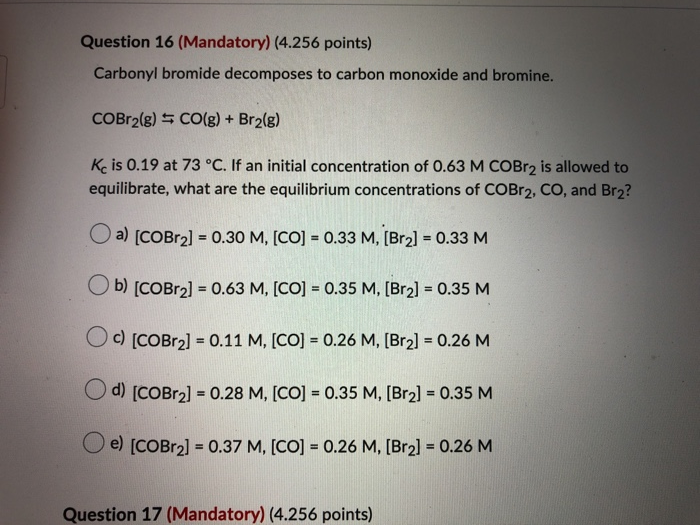Question 16 (Mandatory) (4.256 points) Carbonyl bromide decomposes to carbon monoxide and bromine. COBr2(g) = CO(g) + Br2(g) Kc is 0.19 at 73 °C. If an initial concentration of 0.63 M COBr2 is allowed to equilibrate, what are the equilibrium concentrations of COBr2, CO, and Br2? Oa) (COBr2] = 0.30 M, [CO] = 0.33 M, (Bra] = 0.33 M Ob) (COBr2] = 0.63 M, [CO] = 0.35 M, (Br2] = 0.35 M Oc) [COBr2] = 0.11 M, [CO] = 0.26 M, (Br2] = 0.26 M d) [COBr2] = 0.28 M, [CO] = 0.35 M, (Br2] = 0.35 M e) (COBr2] = 0.37 M, [CO] = 0.26 M, (Br2] = 0.26 M Question 17 (Mandatory) (4.256 points)

Correct option is : (e) [COBr2] = 0.37 M, [CO] = 0.26 M, [Br2] = 0.26 M

Explanation

Given : initial concentration of COBr2 = 0.63 M

Kc = 0.19

 ICE table COBr2 (g)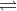CO (g) Br2 (g) Initial conc. 0.63 M 0 0 Change -x +x +x Equilibrium conc. 0.63 M - x +x +x

Kc = [CO]eq[Br2]eq / [COBr2]eq

0.19 = [(x) * (x)] / (0.63 M - x)

Solving for x, x = 0.264 M

[CO]eq = [Br2]eq = x = 0.26 M

[COBr2]eq = 0.63 M - x

[COBr2]eq = 0.63 M - 0.26 M

[COBr2]eq = 0.37 M

#### Earn Coin

Coins can be redeemed for fabulous gifts.

Similar Homework Help Questions
• ### Carbonyl bromide decomposes to carbon monoxide and bromine. COBr2(g) = CO(g) + Br2(g) Kc is 0.190 at 73 °C. If you plac...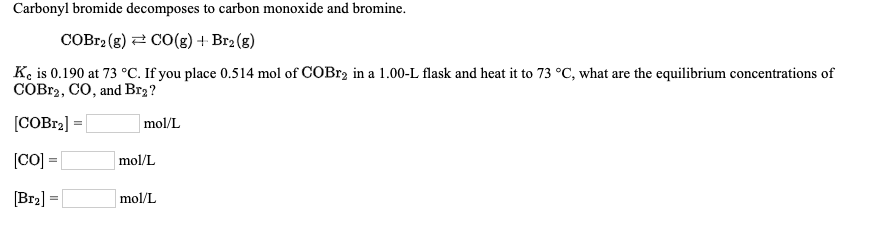Carbonyl bromide decomposes to carbon monoxide and bromine. COBr2(g) = CO(g) + Br2(g) Kc is 0.190 at 73 °C. If you place 0.514 mol of COBr2 in a 1.00-L flask and heat it to 73 °C, what are the equilibrium concentrations of COBr2, CO, and Br? [COBr2] = mol/L [CO] = mol/L [Bry] = mol/L The equilibrium constant for the dissociation of iodine molecules to iodine atoms 12(g) = 2 (g) is 3.76 x 10-3 at 1000 K. Suppose 0.338...

• ### Question 20 Carbonyl bromide decomposes to carbon monoxide and bromine according to the following reaction. COBr2(g)...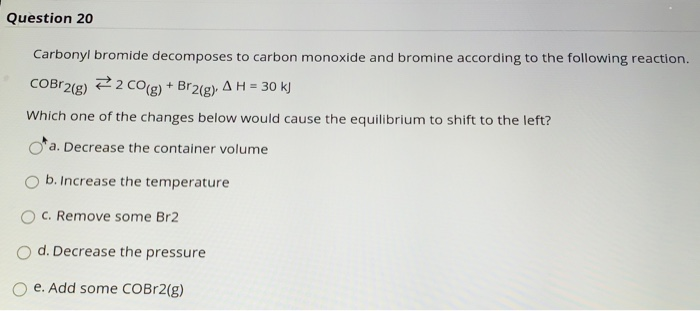Question 20 Carbonyl bromide decomposes to carbon monoxide and bromine according to the following reaction. COBr2(g) e2 CO(g) + Br2(g). A H = 30 kJ Which one of the changes below would cause the equilibrium to shift to the left? o'a. Decrease the container volume b. Increase the temperature C. Remove some Br2 d. Decrease the pressure e. Add some COBr2(g) Question 19 Find the pH of a 0.135 M aqueous solution of hypobromous acid (HOBr), for which Ka =...

• ### Equal amounts of carbon monoxide and bromine react at 70° C to form carbonyl bromide. CO...

Equal amounts of carbon monoxide and bromine react at 70° C to form carbonyl bromide. CO (g) + Br2 (g) <-->COBr2 (g) a) What is the equilibrium constant Kp at this temperature of the reaction when there is 47.2 volume-% of carbon monoxide in the equilibrium mixture and the total pressure in the equilibrium mixture is 1.0 MPa? Give the answer to four decimal places. The balance equation unit does not need to be written into the answer. (use partial...

• ### A more challenging blue-numbered questions answered in Appendix R 749 2 at *17. Carbonyl bromide decomposes...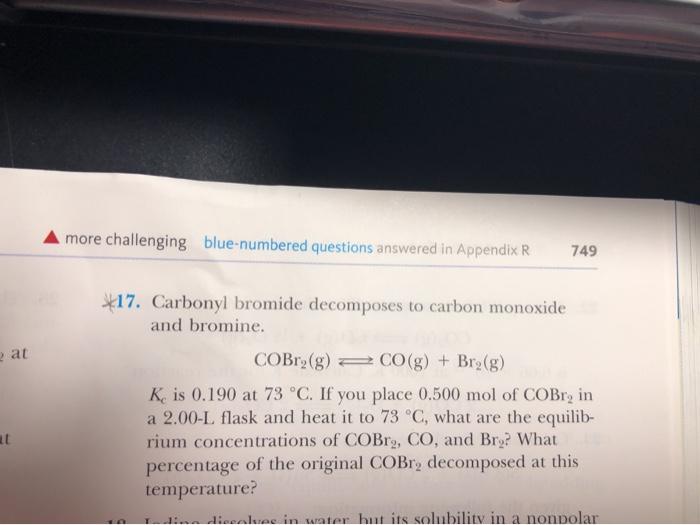A more challenging blue-numbered questions answered in Appendix R 749 2 at *17. Carbonyl bromide decomposes to carbon monoxide and bromine. COBr2(g) 2 CO(g) + Br2(g) K is 0.190 at 73 °C. If you place 0.500 mol of COBro in a 2.00-L flask and heat it to 73 °C, what are the equilib- rium concentrations of COBr, CO, and Bry? What percentage of the original COBr, decomposed at this temperature? L i nkuee in water but its solubility in a...

• ### 26. A mixture 0.500 mole of carbon monoxide and 0.400 mole of bromine was placed into a rigid 1.00-L container and...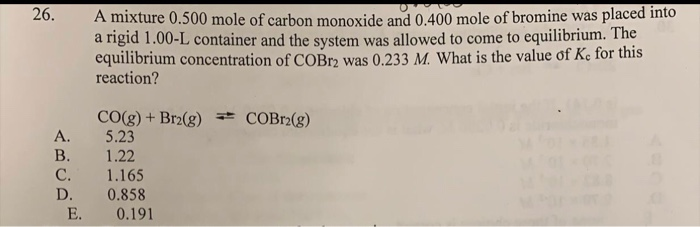26. A mixture 0.500 mole of carbon monoxide and 0.400 mole of bromine was placed into a rigid 1.00-L container and the system was allowed to come to equilibrium. The equilibrium concentration of COBr) was 0.233 M. What is the value of Kc for this reaction? COBr2(g) CO(g) + Br2(g) + 5.23 1.22 1.165 0.858 0.191 E.

• ### 26. A mixture 0.500 mole of carbon monoxide and 0.400 mole of bromine was placed into...26. A mixture 0.500 mole of carbon monoxide and 0.400 mole of bromine was placed into a rigid 1.00-L container and the system was allowed to come to equilibrium. The equilibrium concentration of COBr) was 0.233 M. What is the value of Kc for this reaction? COBr2(g) CO(g) + Br2(g) + 5.23 1.22 1.165 0.858 0.191 E. INSTRUCTIONS: Answer all questions in the question paper provided. SECTION A (Multiple choice) 1. Consider the general reaction 5Br (aq) + BrO; (aq)...

• ### STATISTICS 1200 FORMULAS (Besides writing your name, marking on this packet is NOT allowed (even if...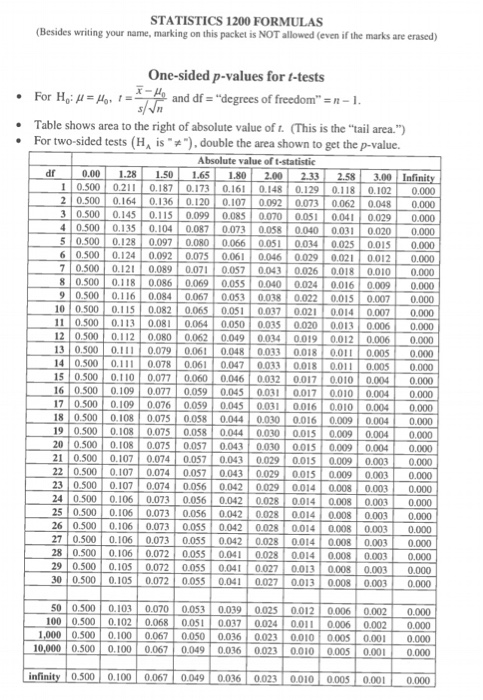STATISTICS 1200 FORMULAS (Besides writing your name, marking on this packet is NOT allowed (even if the marks are erased) One-sided p-values for t-tests • For H: 4= Hon t = * - file and df ="degrees of freedom" =n-1. s/n Table shows area to the right of absolute value of t. (This is the "tail area.") • For two-sided tests (H, is""), double the area shown to get the p-value. Absolute value of t-statistic 2.33 --- dr 0.00 1.28...